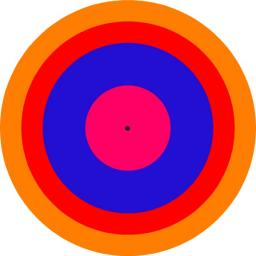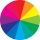# Perimeter to area

Calculate the area of a circle with a perimeter of 15 meters.

S =  17.9049 m2

### Step-by-step explanation:Did you find an error or inaccuracy? Feel free to write us. Thank you!#### You need to know the following knowledge to solve this word math problem:

We encourage you to watch this tutorial video on this math problem:

## Related math problems and questions:

• ShapePlane shape has a maximum area 677 mm2. Calculate its perimeter if perimeter is the smallest possible.
• CircleHow big is an area of a circle if its circumference is 51.2 cm?
• Area of a circleThe circumference of the circle is 150.72 centimeters. Calculate the area of the circle.
• Area to perimeterCalculate circle circumference if its area is 254.34cm2
• Perimeter of the circleCalculate the perimeter of the circle in dm, whose radius equals the side of the square containing 0.49 dm2?Calculate the radius of the quadrant, which area is equal to area of circle with radius r = 15 cm.Determine the radius of the circle if its perimeter and area is the same number.
• Circle - simpleThe circumference of a circle is 198 mm. How long in mm is its diameter?
• Four circles1) Calculate the circle radius if its area is 400 cm square 2) Calculate the radius of the circle whose circumference is 400 cm. 3) Calculate circle circumference if its area is 400 cm square 4) Calculate the circle's area if perimeter 400 cm.Calculate the radius of the circle whose length is 107 cm larger than its diameter
• 30-gonAt a regular 30-gon the radius of the inscribed circle is 15cm. Find the "a" side size, circle radius "R", circumference, and content area.
• Square s3Calculate the diagonal of the square, where its area is 0.49 cm square. And also calculate its circumference.Find the radius of the circle with area S = 200 cm².The circle has a radius 6 cm. Calculate:Circumference of a circle is 6.28. What is the area of the circle?Calculate the circumference of a quarter circle if its content is S = 314 cm2.Alley measured a meters. At the beginning and end are planted poplar. How many we must plant poplars to get the distance between the poplars 15 meters?